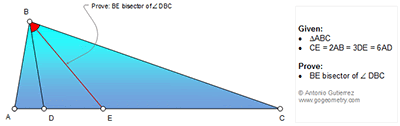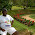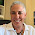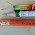## Thursday, November 12, 2015

### Geometry Problem 1164: Triangle, Angle Bisector, Cevian

Geometry Problem. Post your solution in the comments box below.
Level: Mathematics Education, High School, Honors Geometry, College.

Click the diagram below for more details.#### 7 comments:

1.Let AD=x so DE=2x, EC=6x and AB=3x
calculate BD and BC using cosine formula in triangles ABD and ABC .we get
BD^2=10x^2-6x^2.cos(A)
BC^2=90x^2-54x^2.cos(A)
(BD/BC)^2= 1/9 => BD/BC=1/3= ED/EC
so BE bisect angle DBC

2.Let AD = p so that DE = 2p, CE = 6p and AB = 3p

Now AB^2 = 9p^2 = AD.AC so AB is tangential to DBC at B

From similar Tr.s AD/AB = BD/BC = 1/3 = 2p/6p = DE/EC

Hence BE bisects < DBC

Sumith Peiris
Moratuwa
Sri Lanka

3.Tr BAE isoceles => ang ABE=ang AEB => ABD + DBE = EBC + ECB
=> DBE = EBC ( ABD = ECB )

1.Excellent simple solution!

2.Yes, but why ¿ABD=ECB?, because:
The triangle ▲ABD is proportional to the triangle ▲BCA
BAC = BAD (They have in common the angle A)
Its sides are proportional:AC/AB=AB/AD=3

4.See relations between tang and secant
Ang ABD = 1/2 arc BD, Ang ACB = 1/2 arc BD
or Tr ABD similar to Tr ABC

5.Use Stewarts Law with triangle ABC and the cevian BD:

AB = 3x, AD = x, DE = 2x, CE = 6x

(3x)^2*8x + BC^2*x = 9x(BD^2 + 2x^2)
That simplifies to BC^2 = 9BD^2 or BC = 3BD

Since BC:BD = 3:1 and CE:DE = 3:1 also BE is the angle bisector by the angle bisector theorem.